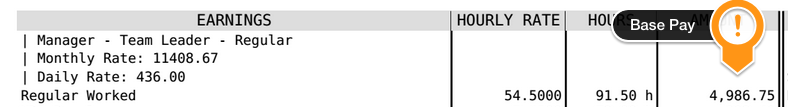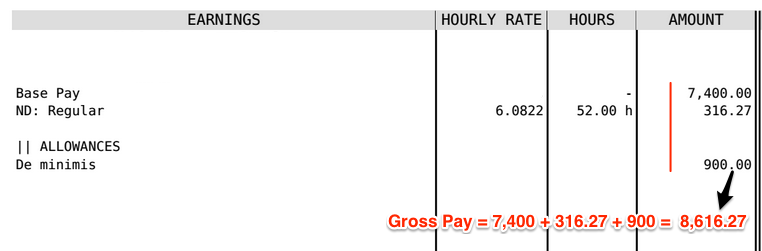# Terms Used When Calculating Payroll

The Financial industry has a few terms used when calculating payroll that you should be aware of:

Base Pay: This is a persons Salary or Wage. This is constant for a Monthly Employee and for an hourly employee it would be calculated by multiplying their rate by hours worked.Gross Pay: The gross pay is the total amount earned by the employee during the pay-period. This would include the Base Pay plus any adjustments (like tardiness, undertime, absences, overtime, ND, holiday and premium pay), bonuses and allowances.Taxable Income: After calculating the gross pay you subtract any non-taxable income (cost of living allowance and de minimis benefits) and allowable/statutory deductions ( SSS, pag-ibig and philhealth).

Tax Payable: Using the Taxable income and the tax table (available here) you can calculate the amount of Tax payable.

Net Pay: Subtract Tax Payable, statutory deductions and other deductions ( like employee cash advances) from the employee’s Gross Pay to calculate Net Pay. This will be the amount payable to the employee.

Here is the Calculation for Net Pay for the above payslip (Assuming single and no dependants S/ME)